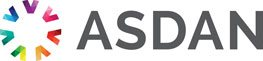# Lifeskills Challenge

## Working with measurement

### Challenge reference: 3111

Notional learning hours 20 1 The learner will develop a basic level of knowledge and skills to enable them to work with time, distance and other common measurements. Please log in to see the rest of this challenge

### Learning outcomes

What the learner needs to know, understand or be able to do

The learner will:

1. Be able to work with time using the 12-hour and 24-hour formats.

2. Be able to work with common units and instruments used in measurement.

### Assessment criteria

What the learner need to demonstrate in order to meet the learning outcome

The learner can:

• Tell the time using the 12- and 24-hour format
• Use straightforward timetables
• Convert different units of time
• Add and subtract times in hours and minutes
• Use common date formats
• Measure length in metric units with commonly used instruments
• Measure weight in metric units
• Read temperatures above and below zero in degrees Celsius
• Measure and compare capacity using metric units